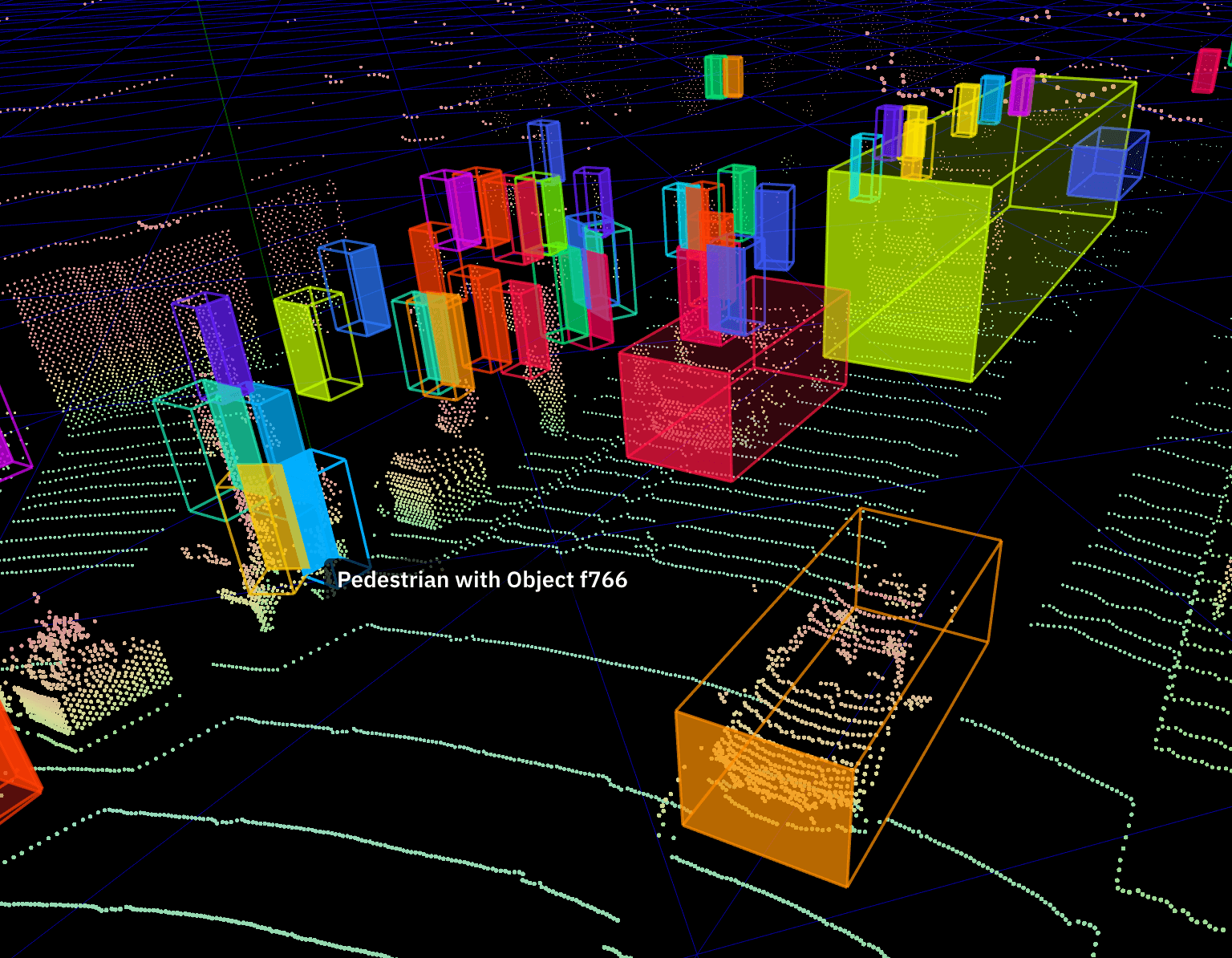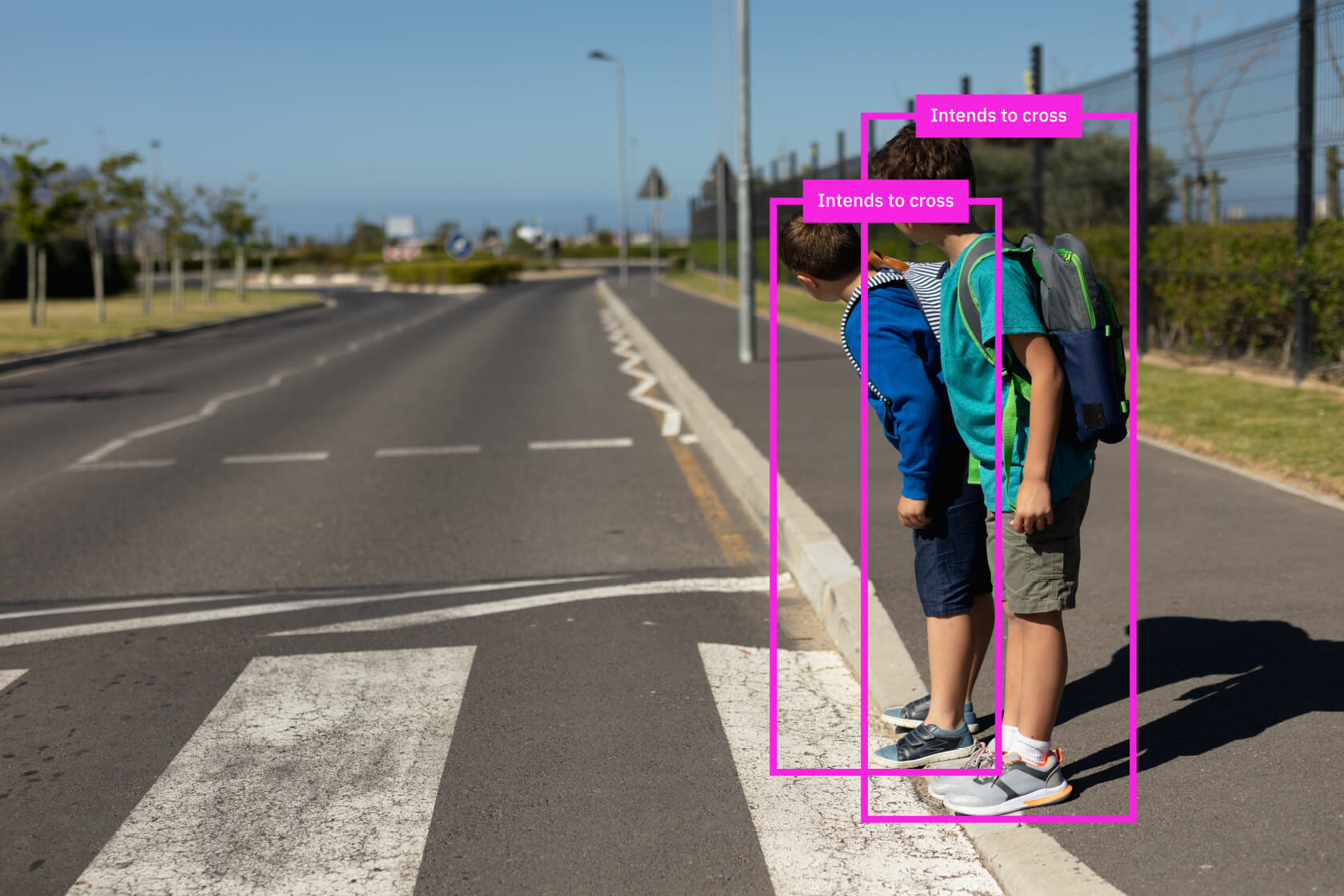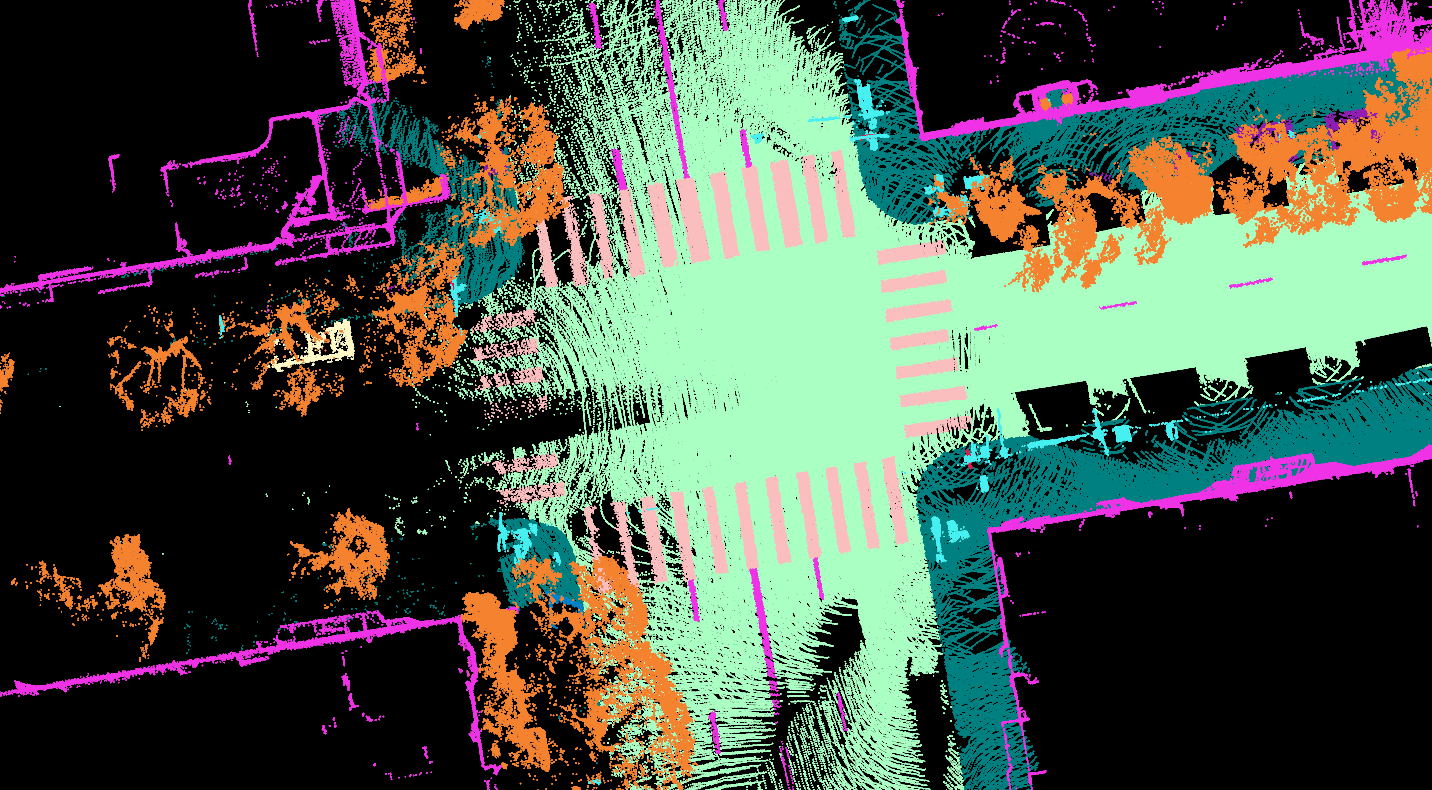# Computer Vision self.__wrap_n=self.__wrap_n||(self.CSS&&CSS.supports("text-wrap","balance")?1:2);self.__wrap_b=(e,n,t)=>{t=t||document.querySelector(`[data-br="\${e}"]`);let r=t.parentElement,o=f=>t.style.maxWidth=f+"px";t.style.maxWidth="";let c=r.clientWidth,a=r.clientHeight,s=c/2-.25,i=c+.5,p;if(c){for(o(s),s=Math.max(t.scrollWidth,s);s+1<i;)p=Math.round((s+i)/2),o(p),r.clientHeight===a?i=p:s=p;o(i*n+c*(1-n))}t.__wrap_o||(typeof ResizeObserver!="undefined"?(t.__wrap_o=new ResizeObserver(()=>{self.__wrap_b(0,+t.dataset.brr,t)})).observe(r):process.env.NODE_ENV==="development"&&console.warn("The browser you are using does not support the ResizeObserver API. Please consider add polyfill for this API to avoid potential layout shifts or upgrade your browser. Read more: https://github.com/shuding/react-wrap-balancer#browser-support-information"))};self.__wrap_n!=1&&self.__wrap_b(":R177n6:",0.4)

•# Detection & Tracking

•# Prediction & Planning

•# Easy to Start, Optimize and Scale self.__wrap_n=self.__wrap_n||(self.CSS&&CSS.supports("text-wrap","balance")?1:2);self.__wrap_b=(e,n,t)=>{t=t||document.querySelector(`[data-br="\${e}"]`);let r=t.parentElement,o=f=>t.style.maxWidth=f+"px";t.style.maxWidth="";let c=r.clientWidth,a=r.clientHeight,s=c/2-.25,i=c+.5,p;if(c){for(o(s),s=Math.max(t.scrollWidth,s);s+1<i;)p=Math.round((s+i)/2),o(p),r.clientHeight===a?i=p:s=p;o(i*n+c*(1-n))}t.__wrap_o||(typeof ResizeObserver!="undefined"?(t.__wrap_o=new ResizeObserver(()=>{self.__wrap_b(0,+t.dataset.brr,t)})).observe(r):process.env.NODE_ENV==="development"&&console.warn("The browser you are using does not support the ResizeObserver API. Please consider add polyfill for this API to avoid potential layout shifts or upgrade your browser. Read more: https://github.com/shuding/react-wrap-balancer#browser-support-information"))};self.__wrap_n!=1&&self.__wrap_b(":R179n6:",0.4)

Label all cars, pedestrians, and cyclists in each frame.

``````1client.createLidarAnnotationTask({
2  instruction: 'Label all cars, pedestrians, and cyclists in each frame.',
3  labels: ['car', 'pedestrian', 'cyclist'],
4  meters_per_unit: 2.3,
5  max_distance_meters: 30
7    // do something with task
8});``````
Run Extraction
•# ML-Powered Data Labeling

•# Automated Quality Pipeline

•# Sensor Agnostic

•# Comprehensive Label Support

••# Best-In-Class Quality self.__wrap_n=self.__wrap_n||(self.CSS&&CSS.supports("text-wrap","balance")?1:2);self.__wrap_b=(e,n,t)=>{t=t||document.querySelector(`[data-br="\${e}"]`);let r=t.parentElement,o=f=>t.style.maxWidth=f+"px";t.style.maxWidth="";let c=r.clientWidth,a=r.clientHeight,s=c/2-.25,i=c+.5,p;if(c){for(o(s),s=Math.max(t.scrollWidth,s);s+1<i;)p=Math.round((s+i)/2),o(p),r.clientHeight===a?i=p:s=p;o(i*n+c*(1-n))}t.__wrap_o||(typeof ResizeObserver!="undefined"?(t.__wrap_o=new ResizeObserver(()=>{self.__wrap_b(0,+t.dataset.brr,t)})).observe(r):process.env.NODE_ENV==="development"&&console.warn("The browser you are using does not support the ResizeObserver API. Please consider add polyfill for this API to avoid potential layout shifts or upgrade your browser. Read more: https://github.com/shuding/react-wrap-balancer#browser-support-information"))};self.__wrap_n!=1&&self.__wrap_b(":R17dn6:",0.4)

### Super Human Quality

3D Sensor Fusion tasks submitted to the platform are first pre-labeled by our proprietary ML-model, then manually reviewed by highly trained workers depending on the ML model confidence scores. All tasks receive additional layers of both human and ML-driven checks.

The resulting accuracy is consistently higher than what a human or synthetic labeling approach can achieve independently.